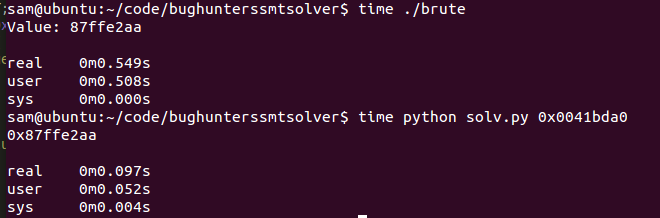# sam-b/z3-stuff

Fetching contributors…Cannot retrieve contributors at this time
68 lines (48 sloc) 3.19 KB

By Sam Brown (@_samdb_)

All the code for this post can be found at: https://github.com/sam-b/z3-stuff/tree/master/a-bug-hunters-constraint-solver

While reading A Bug Hunters Diary the other day I came across a problem the author had in chapter 7 (investigating an XNU kernel bug). The author could control some data which was then used as the address passed to a call instruction. The data went through some basic transforms before it was used as an address, so in order to get it to be an address which the author/attacker controlled they wrote a simple script which brute forced the value they should place in that location as shown below.

```#include <stdio.h>

#define MEMLOC 0x0041bda0
#define SEARCH_START 0x80000000
#define SEARCH_END 0xffffffff

int main(void) {

unsigned int a,b = 0;

for(a = SEARCH_START; a < SEARCH_END; a++ ){
b = (a << 5) + 0x456860;
if(b == MEMLOC){
printf("Value: %08x\n", a);
return 0;
}
}

printf("No valid value found.\n");
return 1;
}
```

This seemed like a perfect opportunity to make use of an SMT solver (see: https://doar-e.github.io/presentations/securityday2015/SecDay-Lille-2015-Axel-0vercl0k-Souchet.html#/ for the best intro I've found), SMT solvers allow us to express 'symbolic' variables and constraints on their values. Then if its possible to find any values which satisfy the constraints, it allows us to access a model which includes possible values which satisfy them. While this particular problem is a trivial example, it is a nice short and simple opportunity to illustrate how to make use of a pretty opaque tool.

In this case I chose to use the Z3 constraint solver/SAT solver from Microsoft, which is open source and has great python bindings. First of we need to import Z3 and read in the target value from the command line:

```from z3 import *
import sys

if __name__ == "__main__":
target = int(sys.argv,16)
```

Next we need to declare a symbolic variable which is equivalent to the 'a' variable in the earlier code, this will be the value which once transformed matches the target value.

```	x = BitVec('x', 32)
```

Now we create a Z3 solver object and add the constraints we know to it, that the value should be between 0x80000000 and 0xffffffff and that when left shifted by 5 with 0x456860 added to it, it should equal the target value.

```	s = Solver()
s.add(target == (x << 5) + 0x456860)
s.add(x > 0x80000000, x < 0xffffffff)
```

Finally we check that our constraints can be satisfied and if so extract the value for x from the model Z3 creates for us.

```	if s.check():
print hex(int(str(s.model()[x])))
else:
print "unsat :("
```

Obviously we could just do the math but that's far less interesting and as you can see below rewriting the brute forcer using z3 saves us a valuable 0.4 seconds :DYou can’t perform that action at this time.